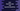# Dart HashSet.take and HashSet.takeWhile methods## How to use HashSet.take and HashSet.takeWhile methods in Dart:

The HashSet class in Dart provides two methods take() and takeWhile() to get a lazy iterable of its elements. Both of these methods are different. This post will show you how to use these methods with examples.

### HashSet.take():

The take method takes one integer value as its parameter and returns one lazy iterable of the first count elements of the HashSet.

The syntax of take is:

``take(int count) → Iterable<E>``
• The count is the only parameter it takes.
• It returns one lazy iterable of the first count elements of the HashSet. We can use this iterable to iterate over the elements.

Let’s learn this method with an example:

``````import 'dart:collection';

void main() {
final givenSet = HashSet.of([1, 2, 3, 4, 5, 6, 7, 8, 9]);
final it = givenSet.take(5);

print('Given HashSet: \${givenSet}');

print('Content of take(5): ');
it.forEach((element) {
print(element);
});
}``````

In this example,

• We created one HashSet of nine numbers. It is assigned to the variable givenSet.
• It used the take method with the count variable as 5 to get the first five values of the HashSet. It is assigned to the it variable.
• We are using forEach on this iterable variable to iterate over the content and print the values.

If you run this program, it will print:

``````Given HashSet: {1, 2, 3, 4, 5, 6, 7, 8, 9}
Content of take(5):
1
2
3
4
5``````

As you can see here, the take(5) method returns the first five elements of the HashSet.

### HashSet.takeWhile:

We have another method takeWhile, which can be used to get a lazy iterable as defined by a given function. Instead of a number, this method takes one function as its parameter and picks the elements defined by that function. Note that this function will pick the elements until it returns false.

The syntax of HashSet.takeWhile is:

``takeWhile(bool fn(E e)) → Iterable<E>``
• It takes one function as its parameter and returns one lazy iterable.
• The function defines the values to pick from the HashSet. It must return one boolean value. The iterable will include the elements till which this function returns true.

Let’s learn how this work with an example:

``````import 'dart:collection';

void main() {
final givenSet = HashSet.of([1, 2, 3, 4, 5, 6, 7, 8, 9]);
final it = givenSet.takeWhile((e) => e < 6);

print('Given HashSet: \${givenSet}');

print('Content of takeWhile: \${it}');
it.forEach((element) {
print(element);
});
}``````
• We are using the same example used before. It will pick all the elements which are smaller than 6. If it finds any number which is equal to or smaller than 6, it will stop.

If you run this program, it will print:

``````Given HashSet: {1, 2, 3, 4, 5, 6, 7, 8, 9}
Content of takeWhile: (1, 2, 3, 4, 5)
1
2
3
4
5``````

As you can see here, it picked all the elements from 1 to 5. Let’s try one more example:

``````import 'dart:collection';

void main() {
final givenSet = HashSet.of([1, 2, 3, 4, 5, 6, 7, 8, 9]);
final it = givenSet.takeWhile((e) => e.isEven);

print('Given HashSet: \${givenSet}');

print('Content of takeWhile: \${it}');
it.forEach((element) {
print(element);
});
}``````

It will give:

``````Given HashSet: {1, 2, 3, 4, 5, 6, 7, 8, 9}
Content of takeWhile: ()``````

As you can see here, it returns an empty iterator because the function is looking for even numbers and since the first number of the hashSet is odd, it will stop the iteration.